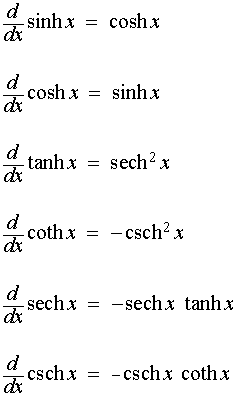Hyperbolic function, its derivative and inverse﻿Hyperbolic function:﻿

In mathematicshyperbolic functions are analogues of the ordinary trigonometric functions, but defined using the hyperbola rather than the circle. Just as the points (cos t, sin t) form a circle with a unit radius, the points (cosh t, sinh t) form the right half of the﻿Sinh(-x)=-sinhx

Cosh(-x)=coshx

Cosh2x+sinh2x=cosh 2x

Sinh2x=2 sinhx.coshx

Cosh(x+y)=coshx.coshy+sinhx.sinhy

Sinh(x+y)=sinhx.coshy+coshx.sinhy﻿